Function Repository Resource:

# KleinBottleGraph

Create a grid graph in the shape of a Klein bottle

Contributed by: Richard Hennigan (Wolfram Research)
 ResourceFunction["KleinBottleGraph"][{a,b}] gives an a×b grid connected as a Graph that corresponds to the 1-skeleton of the Klein bottle. ResourceFunction["KleinBottleGraph"][{a,b,n1,…,nk}] gives a graph corresponding to the product of the Klein bottle and a k-torus.

## Details and Options

ResourceFunction["KleinBottleGraph"] takes the same options as Graph.

## Examples

### Basic Examples (3)

Make a 3×3 Klein bottle graph:

 In:=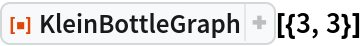Out=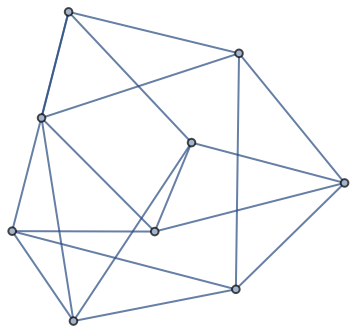Compare to a torus graph:

 In:=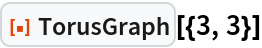Out=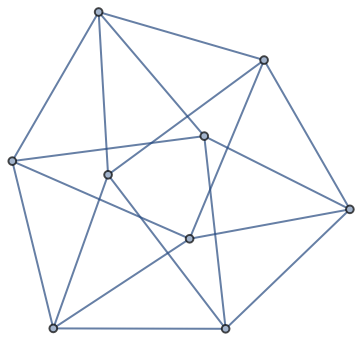Make a 10×20 Klein bottle graph:

 In:=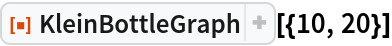Out=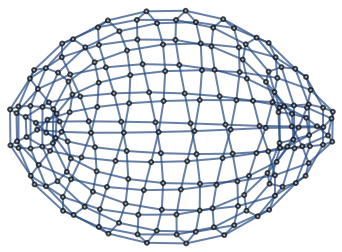Compare to a torus graph:

 In:=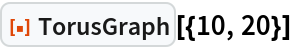Out=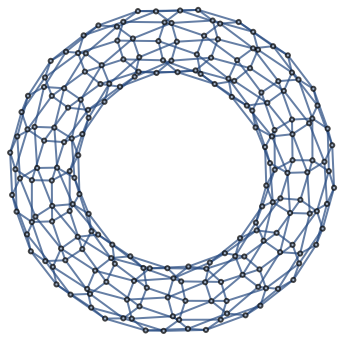The Klein bottle is a torus with a "twist":

 In:=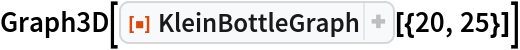Out=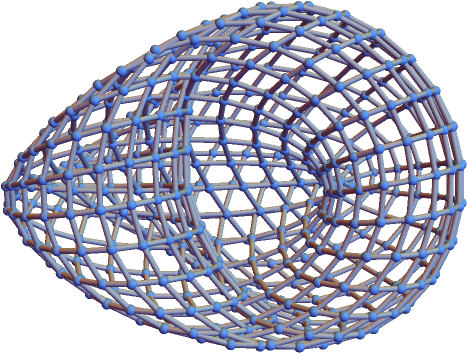Compare to a torus graph:

 In:=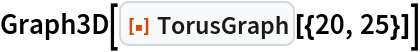Out=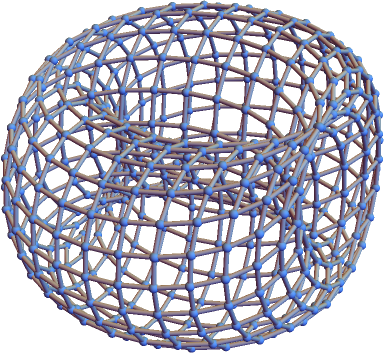### Scope (1)

Make a 10×15×5 Klein bottle graph:

 In:=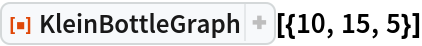Out=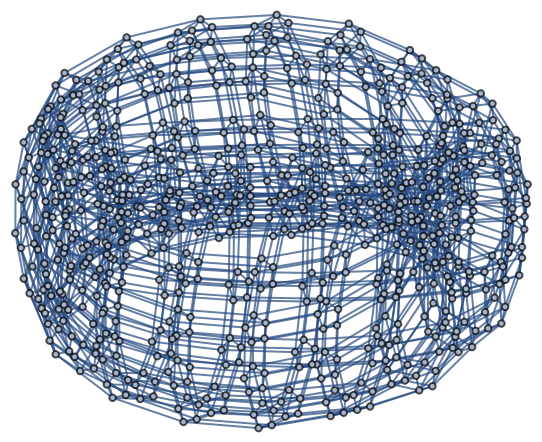### Properties and Relations (4)

Define a torus graph and Klein bottle graph:

 In:=Much like the torus, cutting the Klein bottle in one direction yields a cylinder:

 In:=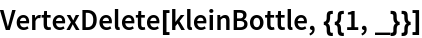Out=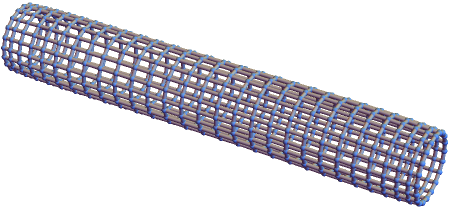In:=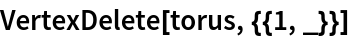Out=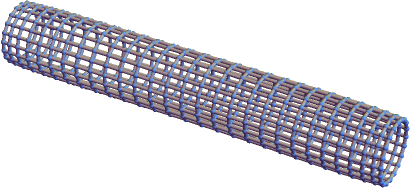However, cutting the Klein bottle in the other direction yields a Möbius strip:

 In:=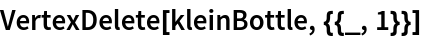Out=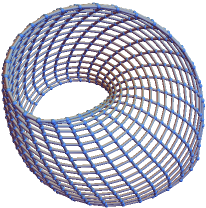The torus still produces a cylinder:

 In:=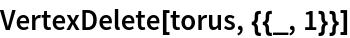Out=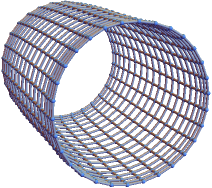In one dimension, KleinBottleGraph is equivalent to TorusGraph:

 In:=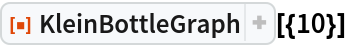Out=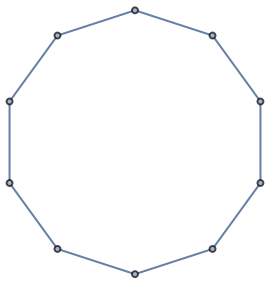In:=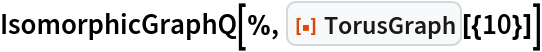Out=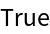Compute the volumes of neighborhoods around a node in the Klein bottle graph:

 In:=Out=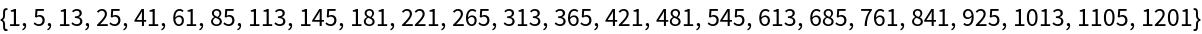Estimate the dimension of the Klein bottle from its graph:

 In:=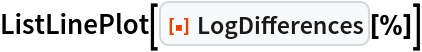Out=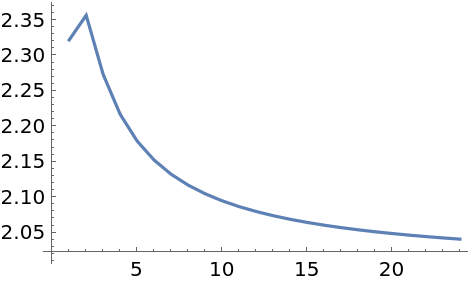This yields the same estimate as the torus:

 In:=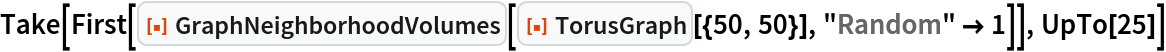Out=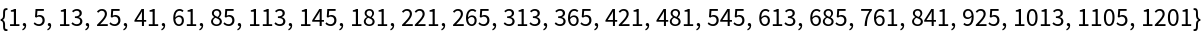In:=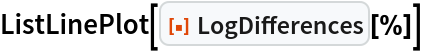Out=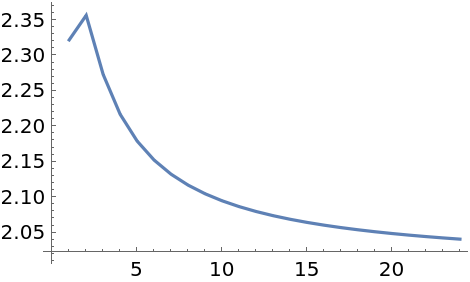Perform a three-way comparison between grid graphs, toroidal grid graphs and Klein bottle graphs:

 In:=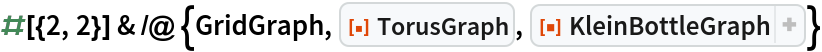Out=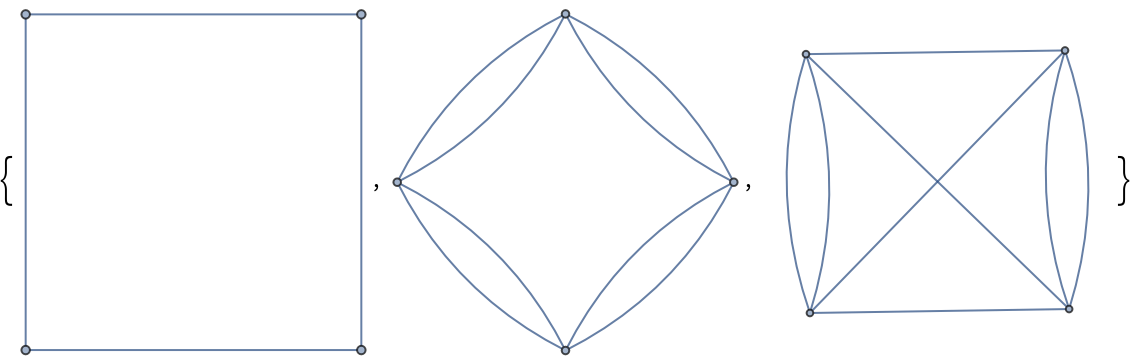In:=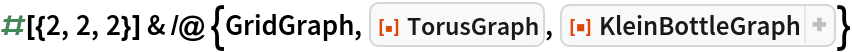Out=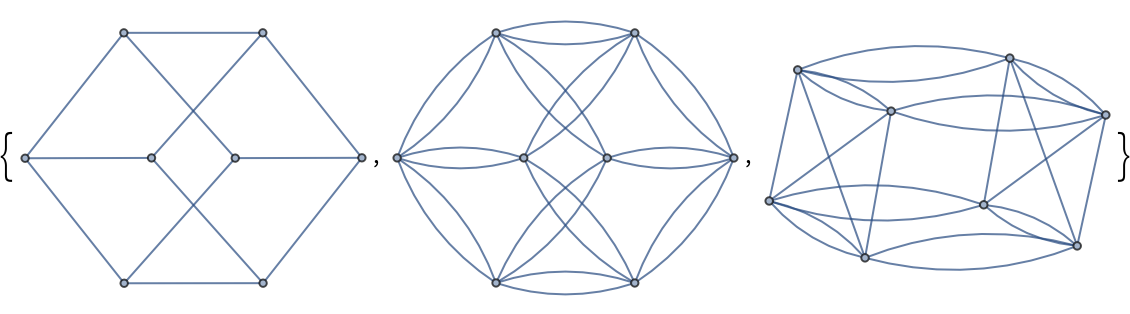In:=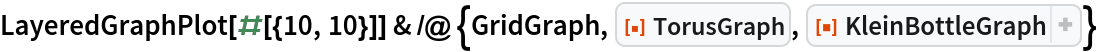Out=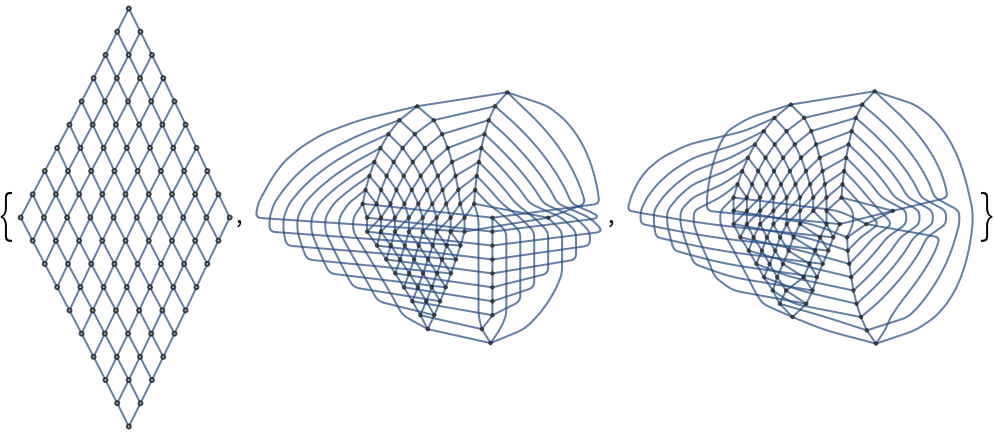### Neat Examples (2)

Show how a ball of radius r grows in the graph when represented as a flat grid (the red edge indicates where the "twist" occurs):

 In:=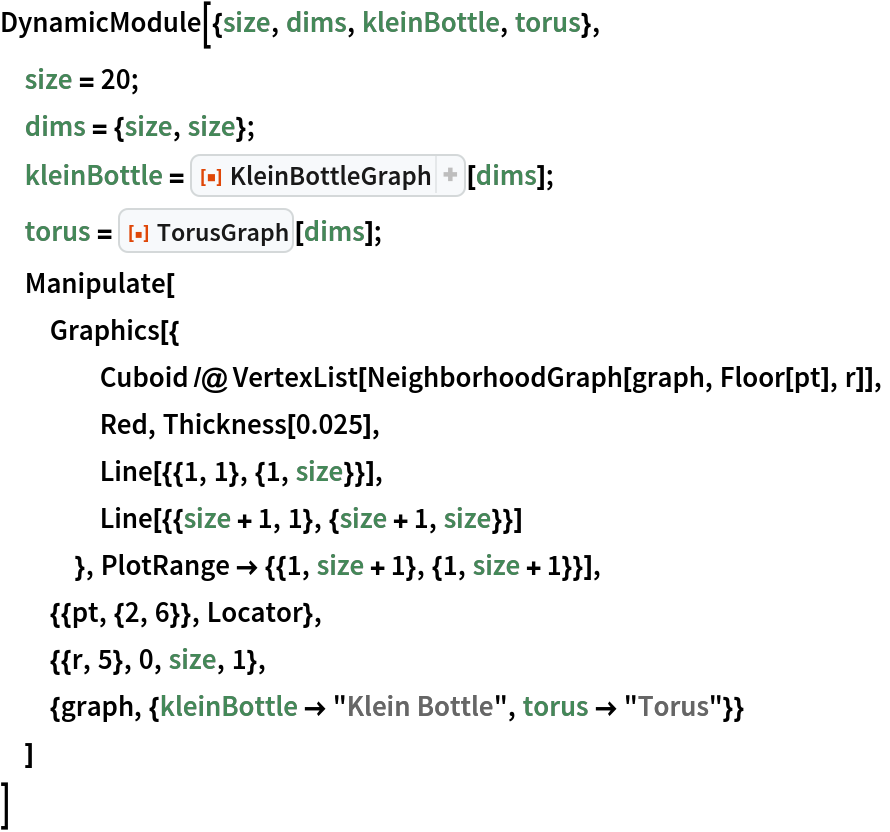Out=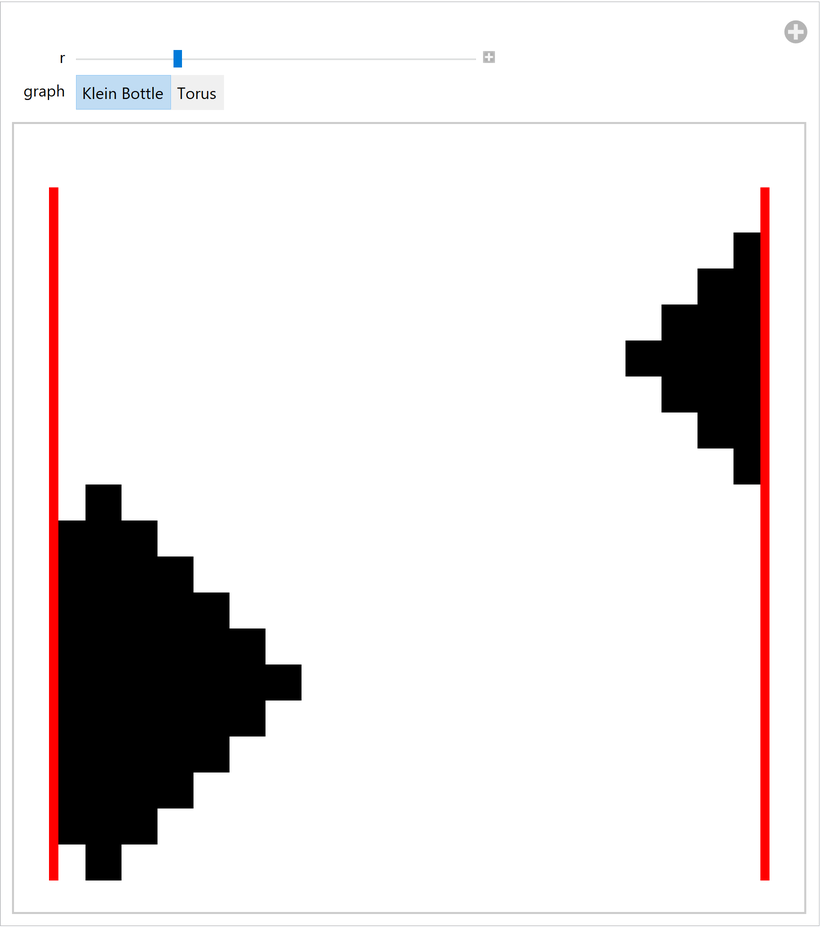Visualize the 3D case:

 In:=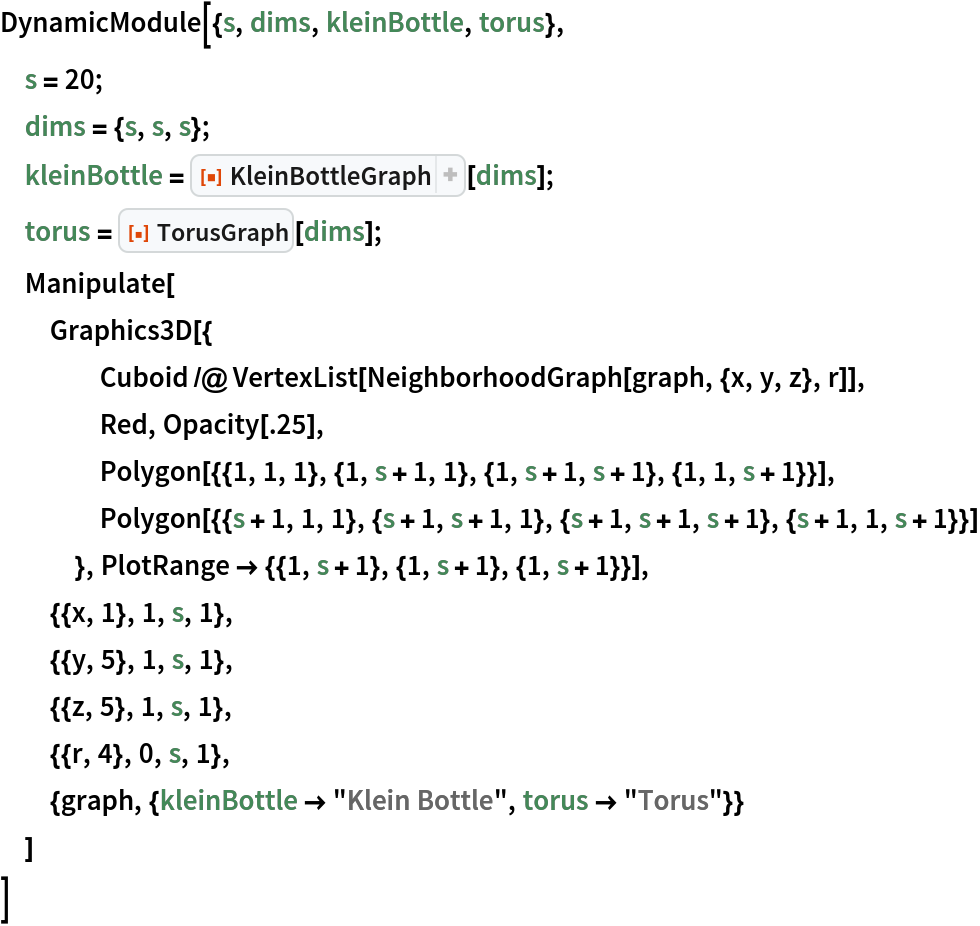Out=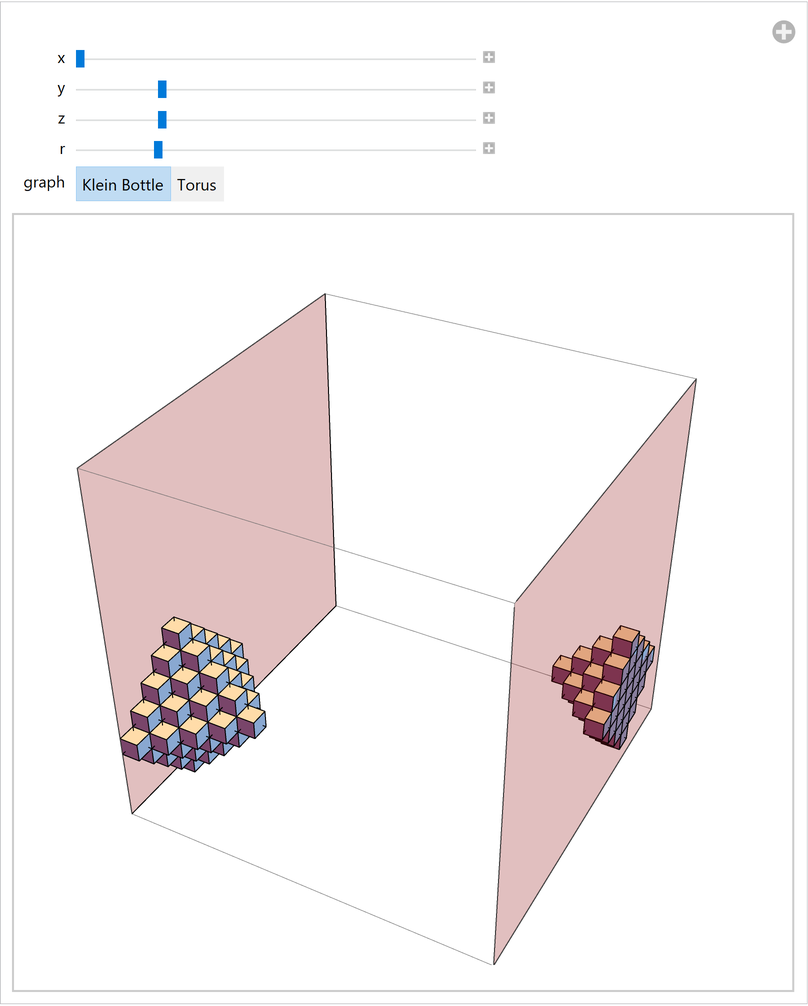## Version History

• 3.0.0 – 28 April 2020
• 1.0.0 – 22 April 2020